# Java流程控制语句（if/switch判断语句和while/do-while/for循环语句）

2021年10月22日
• Java核心
• Java语法基础

“二哥，流程控制语句都有哪些呢？”三妹的脸上泛着甜甜的笑容，她开始对接下来要学习的内容充满期待了，这正是我感到欣慰的地方。

“比如说 if-else、switch、for、while、do-while、return、break、continue 等等，接下来，我们一个个来了解下。”

## # 01、if-else 相关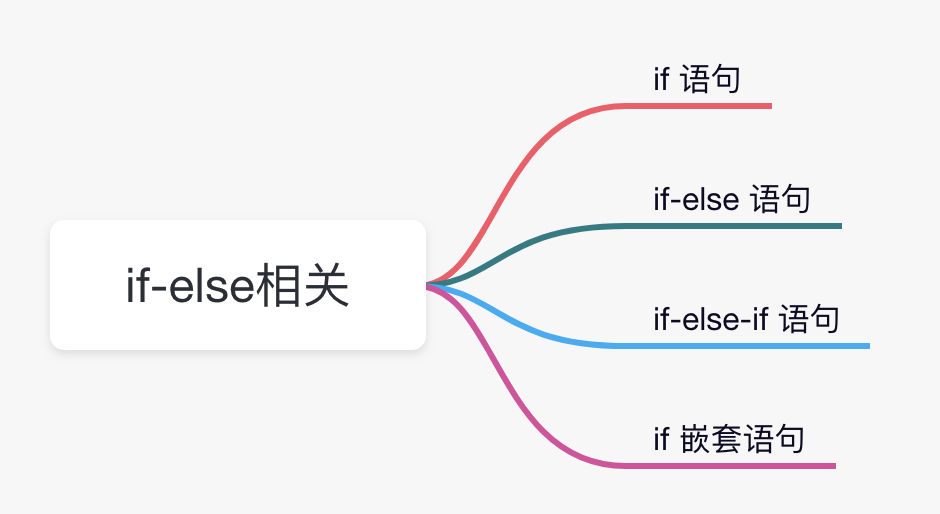1）if 语句

if 语句的格式如下：

``````if(布尔表达式){
// 如果条件为 true，则执行这块代码
}
``````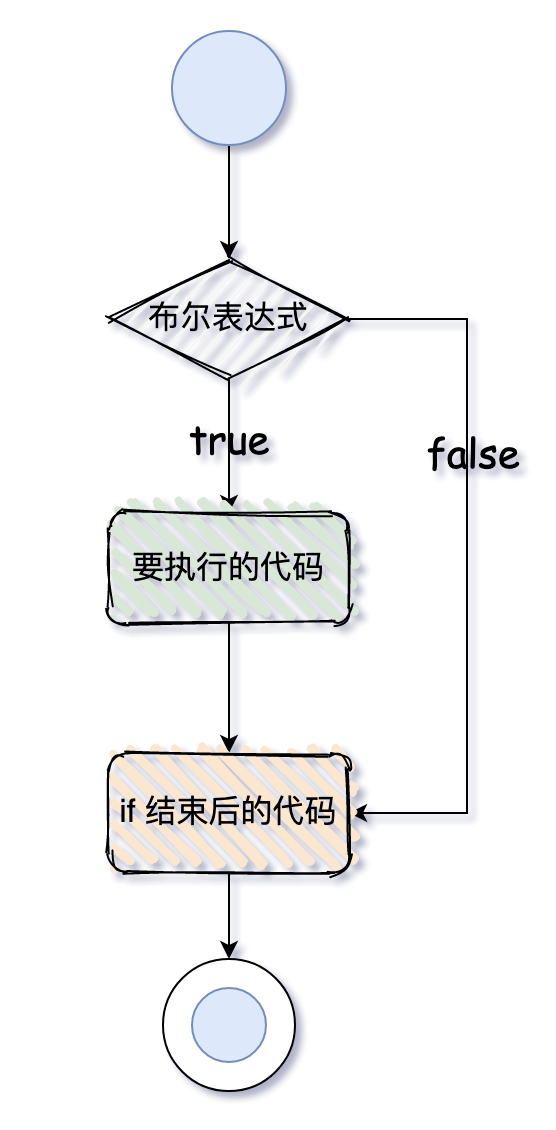``````public class IfExample {
public static void main(String[] args) {
int age = 20;
if (age < 30) {
System.out.println("青春年华");
}
}
}
``````

``````青春年华
``````

2）if-else 语句

if-else 语句的格式如下:

``````if(布尔表达式){
// 条件为 true 时执行的代码块
}else{
// 条件为 false  时执行的代码块
}
``````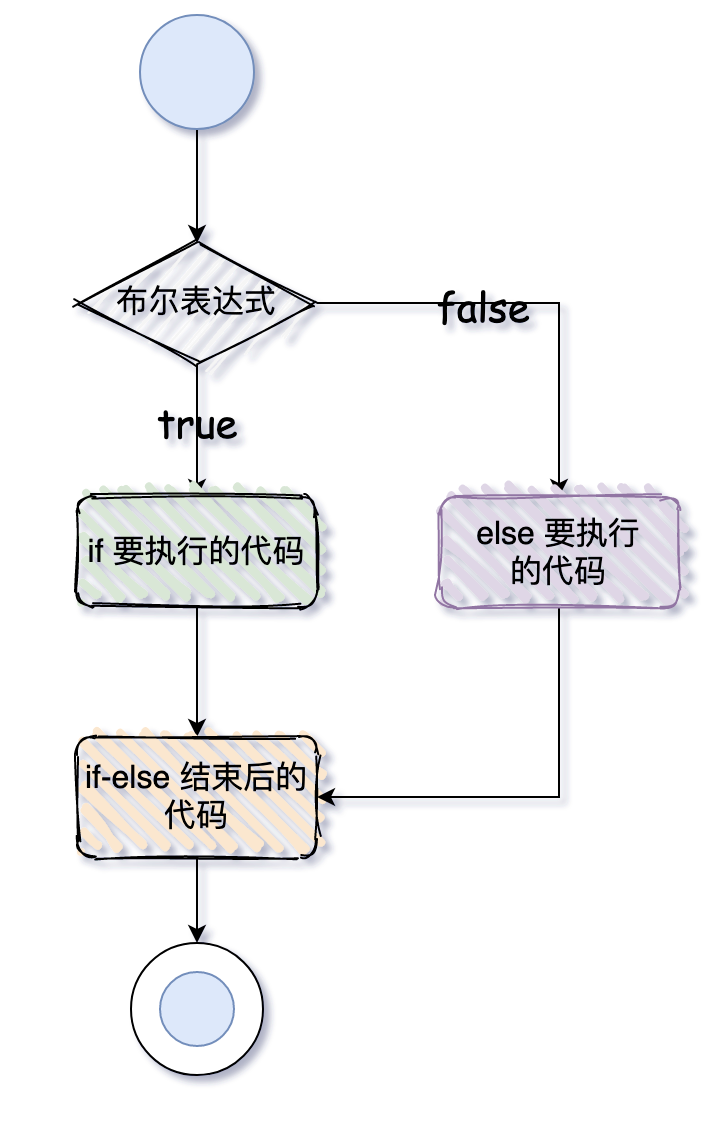``````public class IfElseExample {
public static void main(String[] args) {
int age = 31;
if (age < 30) {
System.out.println("青春年华");
} else {
System.out.println("而立之年");
}
}
}
``````

``````而立之年
``````

``````public class LeapYear {
public static void main(String[] args) {
int year = 2020;
if (((year % 4 == 0) && (year % 100 != 0)) || (year % 400 == 0)) {
System.out.println("闰年");
} else {
System.out.println("普通年份");
}
}
}
``````

``````闰年
``````

``````public class IfElseTernaryExample {
public static void main(String[] args) {
int num = 13;
String result = (num % 2 == 0) ? "偶数" : "奇数";
System.out.println(result);
}
}
``````

``````奇数
``````

3）if-else-if 语句

if-else-if 语句的格式如下：

``````if(条件1){
// 条件1 为 true 时执行的代码
}else if(条件2){
// 条件2 为 true 时执行的代码
}
else if(条件3){
// 条件3 为 true 时执行的代码
}
...
else{
// 以上条件均为 false 时执行的代码
}
````````````public class IfElseIfExample {
public static void main(String[] args) {
int age = 31;
if (age < 30) {
System.out.println("青春年华");
} else if (age >= 30 && age < 40 ) {
System.out.println("而立之年");
} else if (age >= 40 && age < 50 ) {
System.out.println("不惑之年");
} else {
System.out.println("知天命");
}
}
}
``````

``````而立之年
``````

4）if 嵌套语句

if 嵌套语句的格式如下：

``````if(外侧条件){
// 外侧条件为 true 时执行的代码
if(内侧条件){
// 内侧条件为 true 时执行的代码
}
}
``````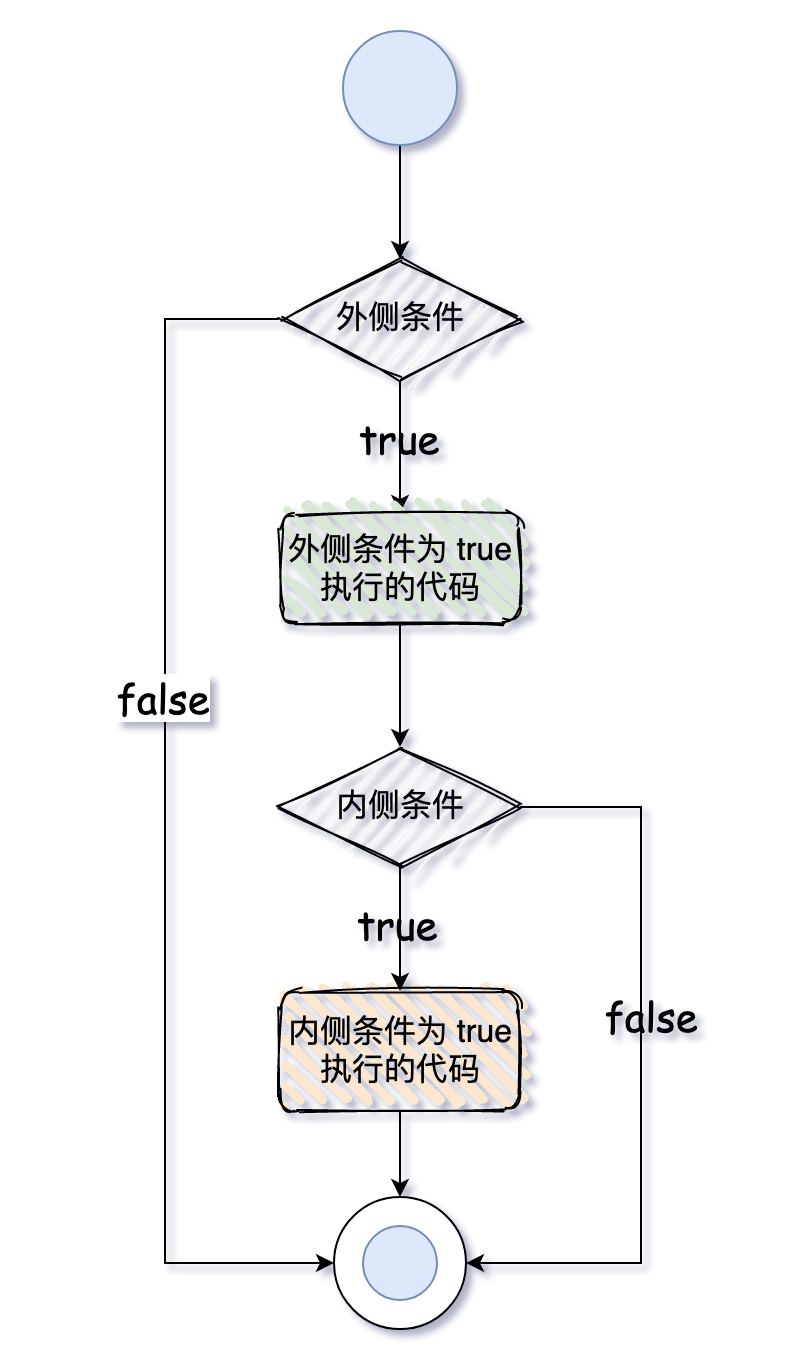``````public class NestedIfExample {
public static void main(String[] args) {
int age = 20;
boolean isGirl = true;
if (age >= 20) {
if (isGirl) {
System.out.println("女生法定结婚年龄");
}
}
}
}
``````

``````女生法定结婚年龄
``````

## # 02、switch 语句

switch 语句用来判断变量与多个值之间的相等性。变量的类型可以是 byte、short、int、long，或者对应的包装器类型 Byte、Short、Integer、Long，以及字符串和枚举。

``````switch(变量) {
case 可选值1:
// 可选值1匹配后执行的代码;
break;  // 该关键字是可选项
case 可选值2:
// 可选值2匹配后执行的代码;
break;  // 该关键字是可选项
......

default: // 该关键字是可选项
// 所有可选值都不匹配后执行的代码
}
``````
• 变量可以有 1 个或者 N 个值。

• 值类型必须和变量类型是一致的，并且值是确定的。

• 值必须是唯一的，不能重复，否则编译会出错。

• break 关键字是可选的，如果没有，则执行下一个 case，如果有，则跳出 switch 语句。

• default 关键字也是可选的。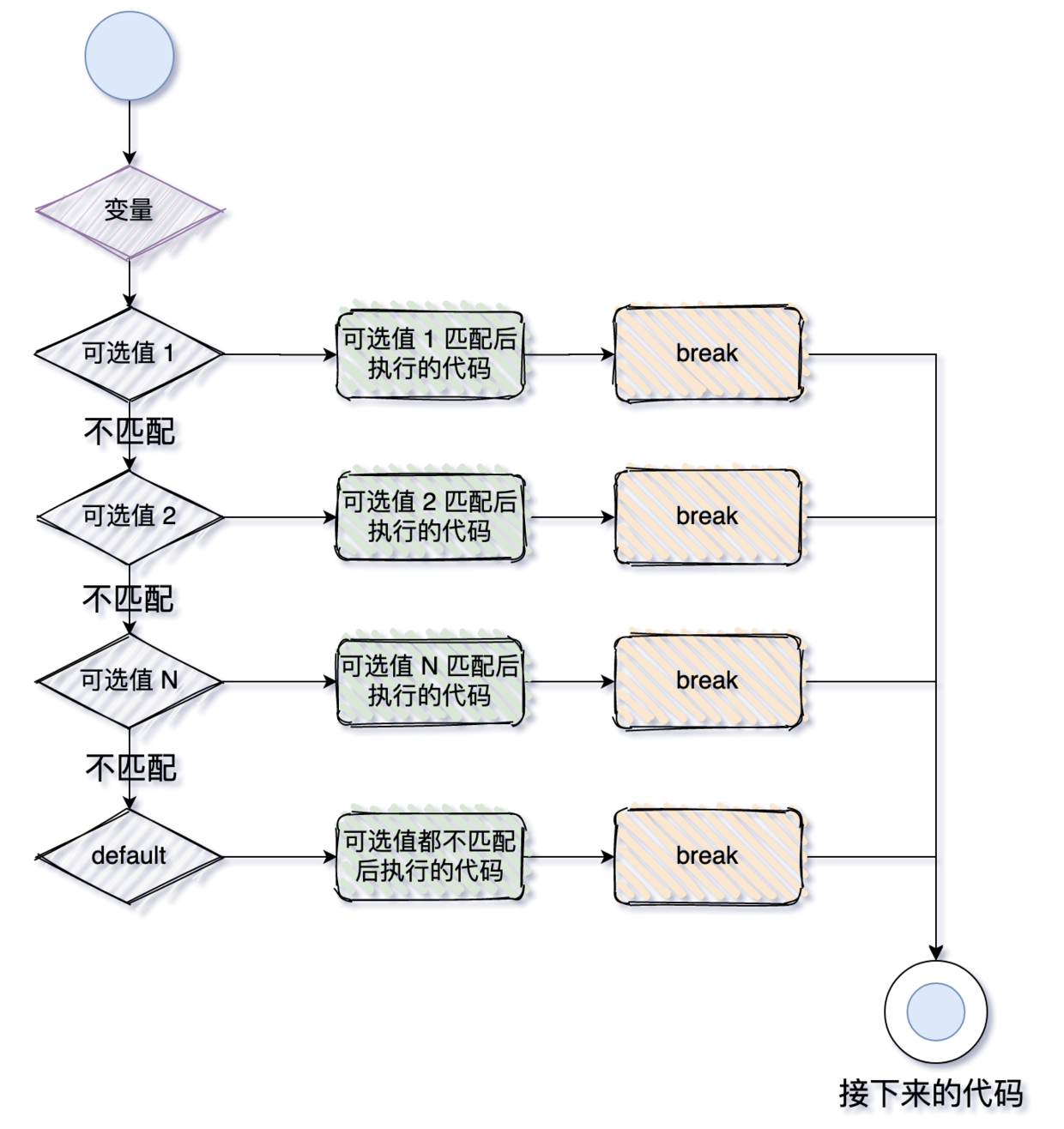``````public class Switch1 {
public static void main(String[] args) {
int age = 20;
switch (age) {
case 20 :
System.out.println("上学");
break;
case 24 :
System.out.println("苏州工作");
break;
case 30 :
System.out.println("洛阳工作");
break;
default:
System.out.println("未知");
break; // 可省略
}
}
}
``````

``````上学
``````

``````public class Switch2 {
public static void main(String[] args) {
String name = "沉默王二";
switch (name) {
case "詹姆斯":
System.out.println("篮球运动员");
break;
case "穆里尼奥":
System.out.println("足球教练");
break;
case "沉默王二":
case "沉默王三":
System.out.println("乒乓球爱好者");
break;
default:
throw new IllegalArgumentException(
"名字没有匹配项");

}
}
}
``````

``````乒乓球爱好者
``````

``````public class SwitchEnumDemo {
public enum PlayerTypes {
TENNIS,
FOOTBALL,
UNKNOWN
}

public static void main(String[] args) {
}

private static String createPlayer(PlayerTypes playerType) {
switch (playerType) {
case TENNIS:
return "网球运动员费德勒";
case FOOTBALL:
return "足球运动员C罗";
return "篮球运动员詹姆斯";
case UNKNOWN:
throw new IllegalArgumentException("未知");
default:
throw new IllegalArgumentException(
"运动员类型: " + playerType);

}
}
}
``````

``````篮球运动员詹姆斯
``````

## # 03、for 循环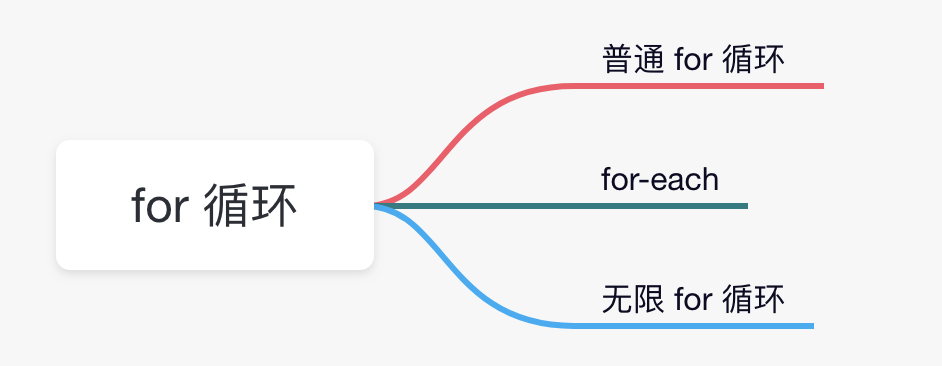1）普通 for 循环

1）初始变量：循环开始执行时的初始条件。

2）条件：循环每次执行时要判断的条件，如果为 true，就执行循环体；如果为 false，就跳出循环。当然了，条件是可选的，如果没有条件，则会一直循环。

3）循环体：循环每次要执行的代码块，直到条件变为 false。

4）自增/自减：初始变量变化的方式。

``````for(初始变量;条件;自增/自减){
// 循环体
}
``````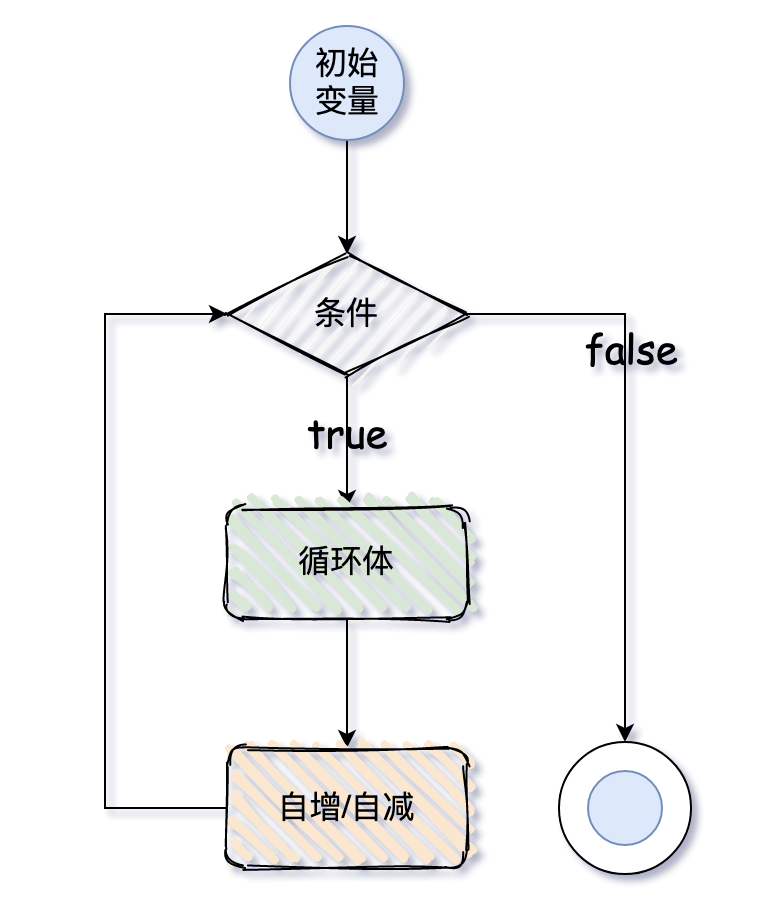``````public class ForExample {
public static void main(String[] args) {
for (int i = 0; i < 5; i++) {
System.out.println("沉默王三好美啊");
}
}
}
``````

``````沉默王三好美啊

``````

“哎呀，二哥，你真的是变着法夸我啊。”

“非也非也，三妹，你看不出我其实在夸我自己吗？循环语句还可以嵌套呢，这样就可以打印出更好玩的呢，你要不要看看？”

“好呀好呀！”

“看好了啊。”

``````public class PyramidForExample {
public static void main(String[] args) {
for (int i = 0; i < 5; i++) {
for (int j = 0;j<= i;j++) {
System.out.print("❤");
}
System.out.println();
}
}
}
``````

``````❤
❤❤
❤❤❤
❤❤❤❤
❤❤❤❤❤
``````

“哇，太不可思议了，二哥。”

“嘿嘿。”

2）for-each

for-each 循环通常用于遍历数组和集合，它的使用规则比普通的 for 循环还要简单，不需要初始变量，不需要条件，不需要下标来自增或者自减。来看一下语法：

``````for(元素类型 元素 : 数组或集合){
// 要执行的代码
}
``````

``````public class ForEachExample {
public static void main(String[] args) {
String[] strs = {"沉默王二", "一枚有趣的程序员"};

for (String str : strs) {
System.out.println(str);
}
}
}
``````

``````沉默王二

``````

“呀，二哥，你开始王哥卖瓜了啊。”

“嘿嘿，三妹，你这样说哥会脸红的。”

3）无限 for 循环

“三妹，你想不想体验一下无限 for 循环的威力，也就是死循环。”

“二哥，那会有什么样的后果啊？”

“来，看看就知道了。”

``````public class InfinitiveForExample {
public static void main(String[] args) {
for(;;){
System.out.println("停不下来。。。。");
}
}
}
``````

``````停不下来。。。。

``````

## # 04、while 循环

``````while(条件){
//循环体
}
``````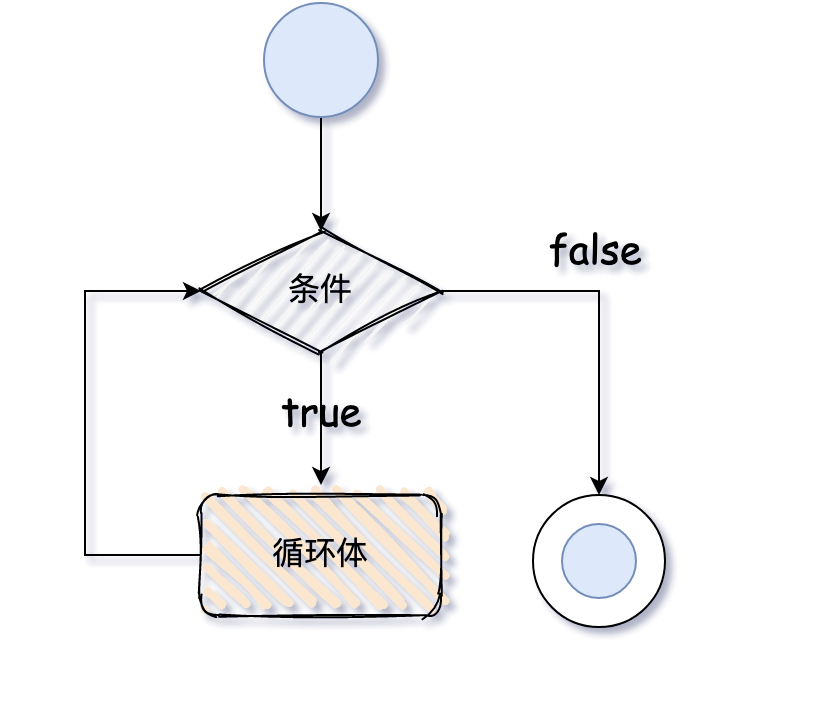``````public class WhileExample {
public static void main(String[] args) {
int i = 0;
while (true) {
System.out.println("沉默王三");
i++;
if (i == 5) {
break;
}
}
}
}
``````

“三妹，你猜猜会输出几次？”

“五次吗？”

“对了，你可真聪明。”

``````沉默王三

``````

“三妹，你想不想体验一下无限 while 循环的威力，也就是死循环。”

“二哥，那会有什么样的后果啊？”

“来，看看就知道了。”

``````public class InfinitiveWhileExample {
public static void main(String[] args) {
while (true) {
System.out.println("停不下来。。。。");
}
}
}
``````

``````停不下来。。。。

``````

## # 05、do-while 循环

``````do{
// 循环体
}while(提交);
``````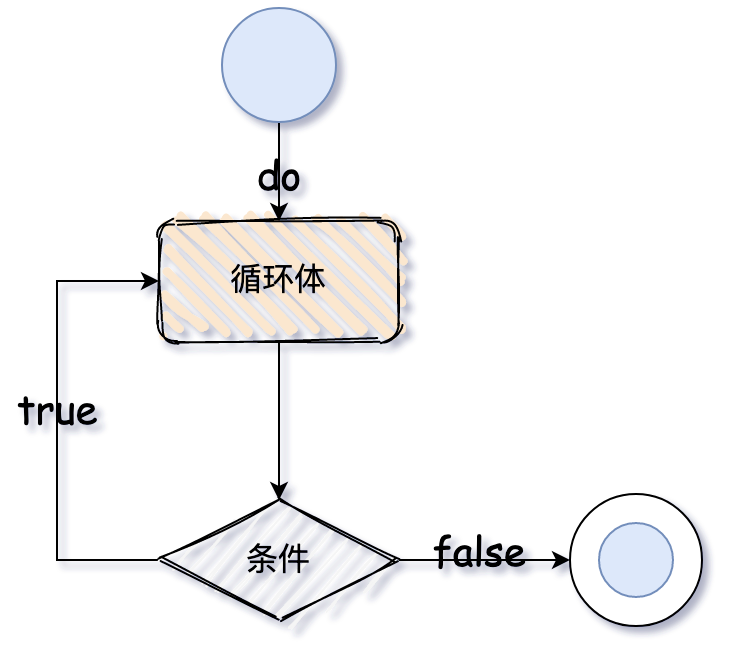``````public class DoWhileExample {
public static void main(String[] args) {
int i = 0;
do {
System.out.println("沉默王三");
i++;
if (i == 5) {
break;
}
} while (true);
}
}
``````

“三妹，你猜猜会输出几次？”

“五次吗？”

“对了，你可真聪明。”

``````沉默王三

``````

“三妹，你想不想体验一下无限 do-while 循环的威力......”

“二哥，又来啊，我都腻了。”

“来吧，例行公事，就假装看看嘛。”

``````public class InfinitiveDoWhileExample {
public static void main(String[] args) {
do {
System.out.println("停不下来。。。。");
} while (true);
}
}
``````

``````停不下来。。。。

``````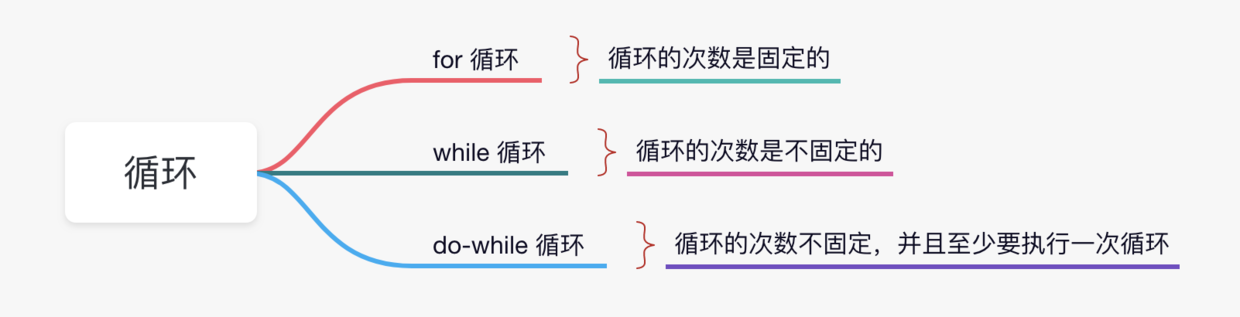## # 06、break

break 关键字通常用于中断循环或 switch 语句，它在指定条件下中断程序的当前流程。如果是内部循环，则仅中断内部循环。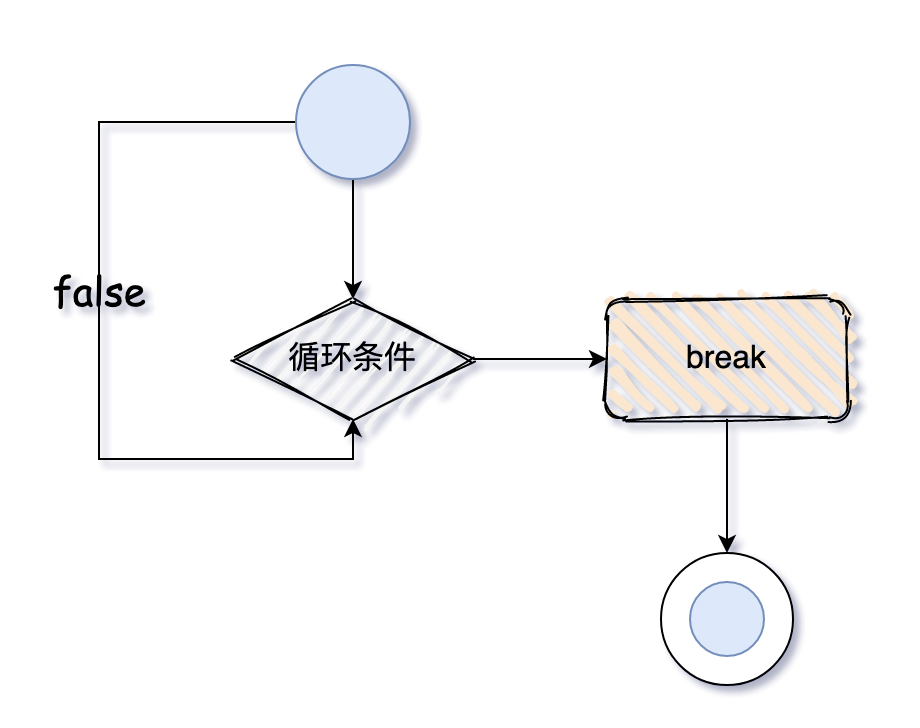``````for (int i = 1; i <= 10; i++) {
if (i == 5) {
break;
}
System.out.println(i);
}
``````

``````for (int i = 1; i <= 3; i++) {
for (int j = 1; j <= 3; j++) {
if (i == 2 && j == 2) {
break;
}
System.out.println(i + " " + j);
}
}
``````

``````int i = 1;
while (i <= 10) {
if (i == 5) {
i++;
break;
}
System.out.println(i);
i++;
}
``````

``````int j = 1;
do {
if (j == 5) {
j++;
break;
}
System.out.println(j);
j++;
} while (j <= 10);
``````

``````switch (age) {
case 20 :
System.out.println("上学");
break;
case 24 :
System.out.println("苏州工作");
break;
case 30 :
System.out.println("洛阳工作");
break;
default:
System.out.println("未知");
break; // 可省略
}
``````

## # 07、continue

``````public class ContinueDemo {
public static void main(String[] args) {
for (int i = 1; i <= 10; i++) {
if (i == 5) {
// 使用 continue 关键字
continue;// 5 将会被跳过
}
System.out.println(i);
}
}
}
``````

``````1
2
3
4
6
7
8
9
10
``````

“二哥，5 真的被跳过了呀。”

“那必须滴。不然就是 bug。”

``````public class ContinueInnerDemo {
public static void main(String[] args) {
for (int i = 1; i <= 3; i++) {
for (int j = 1; j <= 3; j++) {
if (i == 2 && j == 2) {
//  当i=2，j=2时跳过
continue;
}
System.out.println(i + " " + j);
}
}
}
}
``````

``````1 1
1 2
1 3
2 1
2 3
3 1
3 2
3 3
``````

“2 2” 没有输出，被跳过了。

``````public class ContinueWhileDemo {
public static void main(String[] args) {
int i = 1;
while (i <= 10) {
if (i == 5) {
i++;
continue;
}
System.out.println(i);
i++;
}
}
}
``````

``````1
2
3
4
6
7
8
9
10
``````

``````public class ContinueDoWhileDemo {
public static void main(String[] args) {
int i=1;
do{
if(i==5){
i++;
continue;
}
System.out.println(i);
i++;
}while(i<=10);
}
}

``````

``````1
2
3
4
6
7
8
9
10
``````